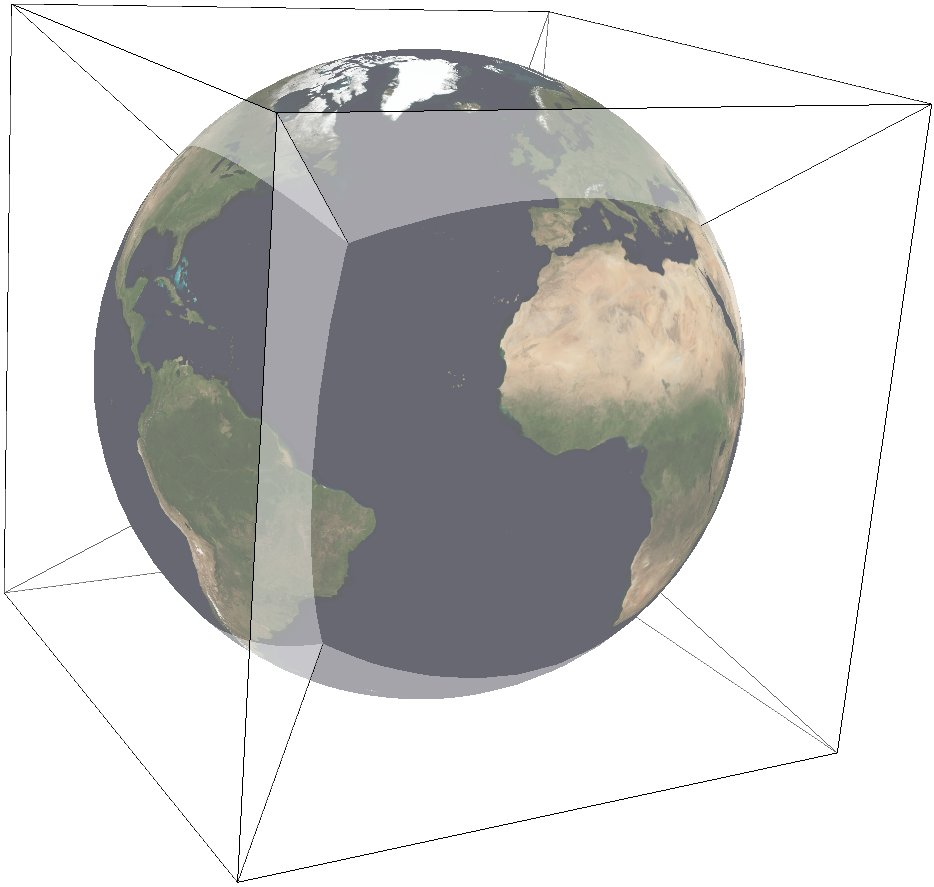# S2¶

 Classification Miscellaneous Available forms Forward and inverse, ellipsoidal Defined area Global Alias s2 Domain 2D Input type Geodetic coordinates Output type Projected coordinates

New in version 8.2.

The S2 projection, like the Quadrilateralized Spherical Cube (QSC) projection, projects a sphere surface onto the six sides of a cube:S2 was created by Google to represent geographic data on the whole earth. The documentation can be found at S2 Geometry It works by first projecting a point on the sphere to a face of the cube. These are called u,v-coordinates, and they are in [-1,1] x [-1,1]. This step is followed by a non-linear transformation to normalize the area of rectangles on the sphere. There are three different choices available for this transformation, meaning that S2 is a family of projections. The final output is in s,t-coordinates, which are in [0,1] x [0,1]. See the comments in S2 Code for an explanation of the tradeoff between speed and area-preservation. Note that the projection is azimuthal when “none” or “linear” is selected for the area-normalization, but it is not azimuthal when “quadratic” or “tangent” is chosen. See S2’s Earthcube page to visualize the unfolded cube and the orientation of each face.

In this implementation, the cube side is selected by choosing one of the following six projection centers:

 `+lat_0=0 +lon_0=0` front cube side `+lat_0=0 +lon_0=90` right cube side `+lat_0=0 +lon_0=180` back cube side `+lat_0=0 +lon_0=-90` left cube side `+lat_0=90` top cube side `+lat_0=-90` bottom cube side

The specific transformation can be chosen with the UVtoST parameter:

 `+UVtoST=linear` fastest, no normalization `+UVtoST=quadratic` fast, good normalization `+UVtoST=tangent` slowest, best normalization `+UVtoST=none` returns u,v-coordinates

Furthermore, this implementation allows the projection to be applied to ellipsoids. A preceding shift to a sphere is performed automatically; see for details. The output of the projection is in s,t-coordinates ([0,1] x [0,1]), so only the eccentricity of the ellipse is taken into account: the absolute value of the axes does not affect the output.

## Usage¶

The following example uses S2 on the right face:

```echo 90 0 | ../bin/proj +proj=s2 +lat_0=0 +lon_0=90 +ellps=WGS84 +UVtoST=linear

0.5 0.5
```

Explanation:

• S2 projection is selected with `+proj=s2`.

• The WGS84 ellipsoid is specified with `+ellps=WGS84`.

• The cube side is selected with `+lat_0=... +lon_0=...`.

• The normalization transformation is selected with `+UVtoST=...`.

## Parameters¶

Note

All parameters for the projection are optional.

+lon_0=<value>

Longitude of projection center.

Defaults to 0.0.

Note

The default convention is to interpret this value as decimal degrees. To specify radians instead, follow the value with the “r” character.

Example: +lon_0=1.570796r

+lat_0=<value>

Latitude of projection center.

Defaults to 0.0.

Note

The default convention is to interpret this value as decimal degrees. To specify radians instead, follow the value with the “r” character.

Example: +lat_0=1.570796r

+ellps=<value>

The name of a built-in ellipsoid definition.

See Ellipsoids for more information, or execute `proj -le` for a list of built-in ellipsoid names.

Defaults to “GRS80”.

+UVtoST=<value>

The area-normalization transformation. Choose from {linear, quadratic, tangent, none}

+x_0=<value>

False easting.

Defaults to 0.0.

+y_0=<value>

False northing.

Defaults to 0.0.